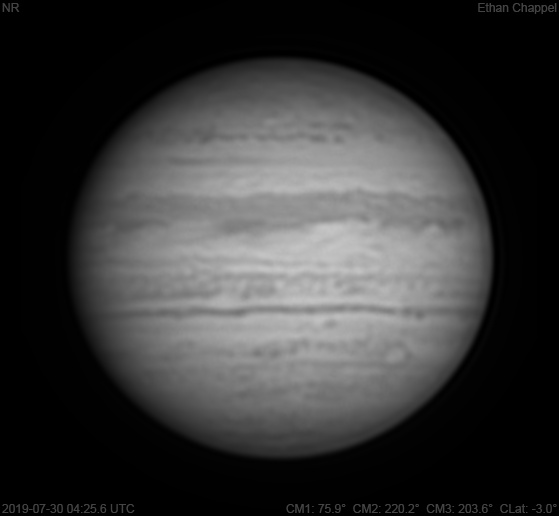# Jupiter 2019-07-30 04:25 UTC

CM1: 75.90°

CM2: 220.20°

CM3: 203.60°

CLat: -3.00°

Description

The last night of imaging in July brought the same good conditions I've seen most of the month.

Notes:

• The North Temperate Belt has a dark streak approaching the central meridian.
• The NEBn has a few small white ovals.
• The EZs has a northern boundary splitting it from the rest of the zone. In visible light, this is where the transition from orange to white occurs.
• The SEBn is mottled.
• The SEBs is dark.
• Oval BA is approaching the eastern limb.

Equipment

ZWO ASI290MM

Celestron EdgeHD 8

Logs
```FireCapture v2.6  Settings
------------------------------------
Observer=Ethan Chappel
Camera=ZWO ASI290MM
Filter=IR
Profile=Jupiter
Diameter=42.83"
Magnitude=-2.43
CMI=76.8° CMII=221.1° CMIII=204.6°  (during mid of capture)
FocalLength=3750mm (F/13)
Resolution=0.16"
Filename=2019-07-30-0426_7-EC-IR-Jup.ser
Date=2019_07_30
Start=04_25_47.769
Mid=04_26_47.772
End=04_27_47.775
Start(UT)=04_25_47.769
Mid(UT)=04_26_47.772
End(UT)=04_27_47.775
Duration=120.006s
Date_format=yyyy_MM_dd
Time_format=HH_mm_ss
LT=UT -6h
Frames captured=7998
File type=SER
Binning=no
Bit depth=8bit
Debayer=no
ROI=400x358
ROI(Offset)=8x8
FPS (avg.)=66
Shutter=15.00ms
Gain=300 (50%)
SoftwareGain=10 (off)
USBTraffic=40 (off)
AutoExposure=off
AutoGain=off
Gamma=50
HighSpeed=off
AutoHisto=75 (off)
FPS=100 (off)
Brightness=1 (off)
Histogramm(min)=0
Histogramm(max)=140
Histogramm=54%
Noise(avg.deviation)=0.73
AutoAlign=false
PreFilter=none
Limit=120 Seconds
Sensor temperature=35.1°C
FireCapture v2.6  Settings
------------------------------------
Observer=Ethan Chappel
Camera=ZWO ASI290MM
Filter=IR
Profile=Jupiter
Diameter=42.83"
Magnitude=-2.43
CMI=75.5° CMII=219.8° CMIII=203.3°  (during mid of capture)
FocalLength=3700mm (F/13)
Resolution=0.16"
Filename=2019-07-30-0424_5-EC-IR-Jup.ser
Date=2019_07_30
Start=04_23_35.529
Mid=04_24_35.534
End=04_25_35.539
Start(UT)=04_23_35.529
Mid(UT)=04_24_35.534
End(UT)=04_25_35.539
Duration=120.010s
Date_format=yyyy_MM_dd
Time_format=HH_mm_ss
LT=UT -6h
Frames captured=7999
File type=SER
Binning=no
Bit depth=8bit
Debayer=no
ROI=400x358
ROI(Offset)=8x8
FPS (avg.)=66
Shutter=15.00ms
Gain=300 (50%)
SoftwareGain=10 (off)
USBTraffic=40 (off)
AutoExposure=off
AutoGain=off
Gamma=50
HighSpeed=off
AutoHisto=75 (off)
FPS=100 (off)
Brightness=1 (off)
Histogramm(min)=0
Histogramm(max)=132
Histogramm=51%
Noise(avg.deviation)=0.73
AutoAlign=false
PreFilter=none
Limit=120 Seconds
Sensor temperature=35.1°C
```